# Geometric Mean (G.M.) Notes | Study Business Mathematics and Logical Reasoning & Statistics - CA Foundation

## CA Foundation: Geometric Mean (G.M.) Notes | Study Business Mathematics and Logical Reasoning & Statistics - CA Foundation

The document Geometric Mean (G.M.) Notes | Study Business Mathematics and Logical Reasoning & Statistics - CA Foundation is a part of the CA Foundation Course Business Mathematics and Logical Reasoning & Statistics.
All you need of CA Foundation at this link: CA Foundation

If a, G, b are in G. P., then G is called the geometric mean between a and b.

If three numbers are in G. P., the middle one is called the geometric mean between the other two.

If a, G1, G2, ..., Gn, b are in G. P.,

then G1, G2, ..., G are called n  G. M.'s between a and b.

The geometric mean of n numbers is defined as the nth root of their product.

Thus if a1, a2, ..., an are n numbers, then their

G. M. = (a1, a2, ... an)1/n

Let G be the G. M. between a and b, then a, G, b are in G. P.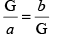or, G2 =ab

or G = √ab

∴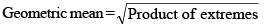Given any two positive numbers a and b, any number of geometric means can be inserted  a1, a2, a3 ..., abe n geometric means between a and b.

then, a1, a2, a3 ..., a,b is a G.P.

Thus, b being the (n + 2)th term, we have

b = arn+1

or, rn+1 = b/a

or,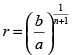Hence,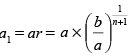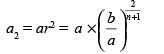.......     .......

......    .......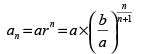Further we can show that the product of these n  G. M.'s is equal to nth power of the single geometric mean between a and b.

Multiplying a1, a2, ... an, we have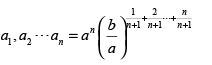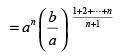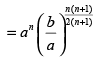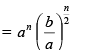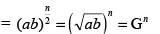= (single G. M. between a and b)n

Example 1. Find the G. M. between 3/2 and 27/2.

Solution: We know that if a is the G. M. between a and b, then

G = √ab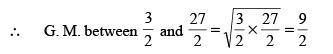Example 2. Insert three geometric means between 1 and 256.

Solution: Let G1, G2, G3, be the three geometric means between 1 and 256.

Then 1, G1, G2, G3, 256 are in G. P.

If r be the common ratio, then t5 = 256

i.e, ar4 = 256 = 1

r4 = 256

or, r2 = 16

or r =  ± 4

When r = 4, G1 = 1. 4 = 4, G2 = 1. (4)2 = 16 and G= 1. (4)3 = 64

When r = – 4, G1 = – 4, G2 = (1) (–4)2 = 16 and G3 = (1) (–4)3 = –64

∴ G.M. between 1 and 256 are 4, 16, 64, or, – 4, 16, –64.

Example 3. If 4, 36, 324 are in G. P. insert two more numbers in this progression so thatit again forms a G. P.

Solution:  G. M. between 4 and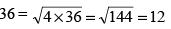G. M. between 36 and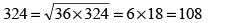If we introduce 12 between 4 and 36 and 108 betwen 36 and 324, the numbers 4, 12, 36, 108, 324 form a G. P.

∴ The two new numbers inserted are 12 and 108.

Example 4. Find the value of n such that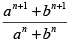may be the geometric mean between a and b.

Solution:  If x be G. M. between a and b, then

x = a1/2.b1/2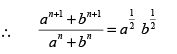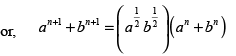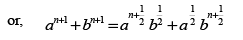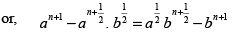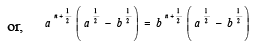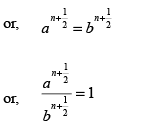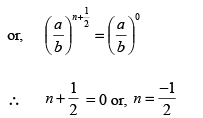The document Geometric Mean (G.M.) Notes | Study Business Mathematics and Logical Reasoning & Statistics - CA Foundation is a part of the CA Foundation Course Business Mathematics and Logical Reasoning & Statistics.
All you need of CA Foundation at this link: CA FoundationUse Code STAYHOME200 and get INR 200 additional OFF

## Business Mathematics and Logical Reasoning & Statistics

81 videos|101 docs|87 tests

Track your progress, build streaks, highlight & save important lessons and more!

,

,

,

,

,

,

,

,

,

,

,

,

,

,

,

,

,

,

,

,

,

;